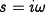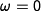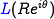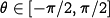# Errata: In the paragraph above Example 9.3, G(*) should be L(*)

For computing the Nyquist plot, it is the loop transfer functionthat should be used. The paragraph should read:
An alternative to computing the Nyquist plot explicitly is to determine the plot from the frequency response (Bode plot), which gives the Nyquist curve for,. We start by plottingfromto, which can be read off from the magnitude and phase of the transfer function. We then plotwithand, which almost always maps to zero. The remaining parts of the plot can be determined by taking the mirror image of the curve thus far (normally plotted using a dashed line). The plot can then be labeled with arrows corresponding to a clockwise traversal around the D contour (the same direction in which the first portion of the curve was plotted).